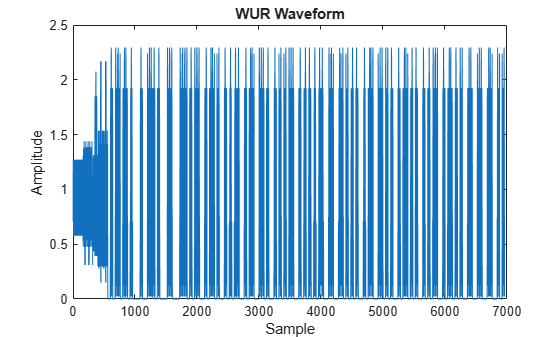# wlanWURConfig

Configure WUR transmission

## Description

The `wlanWURConfig` object is a configuration object for the WLAN wake-up radio (WUR) packet format.

## Creation

### Syntax

``cfgWUR = wlanWURConfig``
``cfgWUR = wlanWURConfig(numSubchannels)``
``cfgWUR = wlanWURConfig(___,Name=Value)``

### Description

example

````cfgWUR = wlanWURConfig` creates a configuration object that initializes transmit parameters for an IEEE® 802.11ba™ WUR PPDU with one 20 MHz subchannel. For a detailed description of the WUR WLAN format, see .```

example

````cfgWUR = wlanWURConfig(numSubchannels)` parameterizes a WUR transmission with the specified number of 20 MHz subchannels. Specify `numSubchannels` as `1`, `2`, or `4`.```

example

````cfgWUR = wlanWURConfig(___,Name=Value)` sets Properties using one or more name-value arguments. For example, `NumTransmitAntennas=3` specifies three transmit antennas.```

## Properties

expand all

Subchannel parameters, specified as a cell array of `wlanWURSubchannel` objects. Each element of the cell array contains properties to configure a 20 MHz subchannel. The default value is a 1-by-1 cell array containing a `wlanWURSubchannel` object with default property values.

Number of transmit antennas, specified as an integer in the interval [1, 8].

Data Types: `double`

Channel bandwidth of the PPDU transmission, returned as one of these values.

• `'CBW20'` — Channel bandwidth of 20 MHz

• `'CBW40'` — Channel bandwidth of 40 MHz

• `'CBW80'` — Channel bandwidth of 80 MHz

Data Types: `char` | `string`

Number of users in the transmission, returned as `1`, `2`, `3`, or `4`.

Data Types: `double`

## Object Functions

 `getActiveSubchannelIndex` Active subchannel indices `getPSDULength` Calculate HE or WUR PSDU length `packetFormat` Return WLAN packet format

## Examples

collapse all

Configure and generate a WLAN waveform containing a WUR packet with default settings.

Parameterize the transmission by creating a WUR configuration object with default property values.

`cfgWUR = wlanWURConfig;`

Generate a PSDU of relevant length.

```psduLength = getPSDULength(cfgWUR); psdu = randi([0 1],8*psduLength,1);```

Generate and plot the waveform.

```waveform = wlanWaveformGenerator(psdu,cfgWUR); figure plot(abs(waveform)) title('WUR Waveform') xlabel('Sample') ylabel('Amplitude')```Create a WUR configuration object, specifying four 20 MHz subchannels.

```numSubchannels = 4; cfgWUR = wlanWURConfig(numSubchannels);```

Configure each 20 MHz subchannel.

```psduLength = [4 8 12 16]; dataRate = {'LDR','HDR','LDR','HDR'}; design = {'Example1','Example2','Example1','Example1'}; cfgSubchannel = cell(1,numSubchannels); psdu = cell(1,cfgWUR.NumUsers); for i = 1:cfgWUR.NumUsers cfgSubchannel{i} = wlanWURSubchannel(PSDULength=psduLength(i), ... DataRate=dataRate{i},SymbolDesign=design{i}); psdu{i} = randi([0 1],8*psduLength(i),1,'int8'); end cfgWUR.Subchannel = cfgSubchannel;```

Specify an oversampling factor of two, and then generate the waveform.

```osf = 2; waveform = wlanWaveformGenerator(psdu,cfgWUR,NumPackets=4, ... IdleTime=1e-5,OversamplingFactor=osf);```

Create a WUR configuration object for a transmission with two subchannels and four transmit antennas.

```numSubchannels = 2; cfgWUR = wlanWURConfig(numSubchannels,NumTransmitAntennas=4);```

Get the active subchannel indices for the transmission.

`idx = getActiveSubchannelIndex(cfgWUR)`
```idx = 1×2 1 2 ```